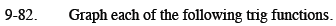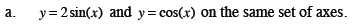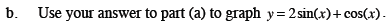Home > CCA2 > Chapter 9 > Lesson 9.3.1 > Problem9-82

9-82.
1. Graph each of the following trig functions. 9-82 HW eTool (Desmos). Homework Help ✎

1. y = 2sin(x) and y = cos(x) on the same set of axes.

2. Use your answer to part (a) to graph y = 2sin(x) + cos(x).Review the Math Notes box in Lesson 7.2.4.$\text{Try adding and then graphing the }y\text{-values of each function for each }\frac{\pi}{2}\text{ multiple of }x.$

Use the eTool below to help you with this problem.
Click on the link at right for the full eTool version: CCA2 9-82 HW eTool.